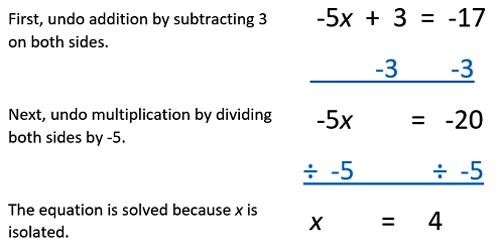Solving an Equation

An equation is a mathematical statement that has two expressions separated by an equal sign. The expression on the left side of the equal sign has the same value as the expression on the right side.

One or both of the expressions may contain variables. Solving an equation means manipulating the expressions and finding the value of the variables.

Examples with explanation:

An example might be: x = 4+8; to solve this equation we would add 4 and 8 and find that x = 12.

An example might be: x – 3 = 5

To keep an equation equal, we must do exactly the same thing to each side of the equation. If we add (or subtract) a quantity from one side, we must add (or subtract) that same quantity from the other side.

to solve this equation we would add 3 to both sides. The equation would become x – 3 + 3 = 5 + 3. This becomes x = 5 + 3 or x = 8.

For example solve the equation: 7x = 21

To keep both sides of an equation equal, we must do exactly the same thing to each side of the equation. If we multiply (or divide) one side by a quantity, we must multiply (or divide) the other side by that same quantity.

In order to solve this equation we would divide both sides by 7. The equation would become 7x/7 = 21/7. When simplified, this would become x = 21/7 or x = 3.

It is possible to substitute the value of x back into the original equation 7*3=21.For example solve the equation: 8x-2=14

To keep both sides of an equation equal, we must do exactly the same thing to each side of the equation. First, add two to each side of the equation so that 8x-2+2=14+2 or 8x=16. If we multiply (or divide) one side by a quantity, we must multiply (or divide) the other side by that same quantity.

In order to solve this equation we would divide both sides by 8. The equation would become 8x/8 = 16/8. When simplified, this would become x = 16/8 or x = 2.

It is possible to substitute the value of x back into the original equation 8*2-2=14.

Information Source: